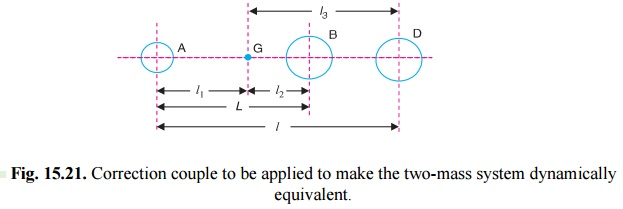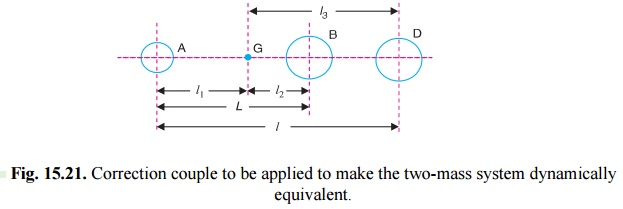Home | | Dynamics of Machines | Correction Couple to be Applied to Make two Mass System Dynamically Equivalent

# Correction Couple to be Applied to Make two Mass System Dynamically EquivalentIn Art. 15.11, we have discussed the conditions for equivalent dynamical system of two bodies. A little consideration will show that when two masses are placed arbitrarily*, then the conditions (i) and (ii) as given in Art. 15.11 will only be satisfied. But the condition (iii) is not possible to satisfy.

CORRECTION COUPLE TO BE APPLIED TO MAKE  TWO MASS SYSTEM DYNAMICALLY EQUIVALENT

In Art. 15.11, we have discussed the conditions for equivalent dynamical system of two bodies. A little consideration will show that when two masses are placed arbitrarily*, then the conditions (i) and (ii) as given in Art. 15.11 will only be satisfied. But the condition (iii) is not possible to satisfy. This means that the mass moment of inertia of these two masses placed arbitrarily, will differ than that of mass moment of inertia of the rigid body.Fig. 15.21. Correction couple to be applied to make the two-mass system dynamically equivalent.

Consider two masses, one at A and the other at D be placed arbitrarily, as shown in Fig

15.21. Let l3  = Distance of mass placed at D from G,

l1= New mass moment of inertia of the two masses;The difference of the torques T' is known as correction couple. This couple must be applied, when the masses are placed arbitrarily to make the system dynamical equivalent. This, of course, will satisfy the condition (iii)

Q. A connecting rod of an I.C. engine has a mass of 2 kg and the distance between the centre of gudgeon pin and centre of crank pin is 250 mm. The C.G. falls at a point 100 m m from the gudgeon pin along the line of centres. The radius of gyration about an axis through the C.G. perpendicular to the plane of rotation is 110 mm. Find the equivalent dynamical system if only one of the masses is located at gudgeon pin.

If the connecting rod is replaced by two masses, one at the gudgeon pin and the other at the crank pin and the angular acceleration of the rod is 23 000 rad/s2 clockwise, determine the correc- tion couple applied to the system to reduce it to a dynamically equivalent system.

Solution. Given : m = 2 kg ; l = 250 mm = 0.25 m ; l1 = 100 mm = 0.1m ; kG = 110 mm = 0.11 m ; Î±= 23 000 rad/s2

Equivalent dynamical system

It is given that one of the masses is located at the gudgeon pin. Let the other mass be located at a distance l2 from the centre of gravity. We know that for an equivalent dynamical system.Correction couple

Since the connecting rod is replaced by two masses located at the two centres (i.e. one at the gudgeon pin and the other at the crank pin), therefore,

l = 0.1 m,      and     l3 = l â€“ l1 = 0.25 â€“ 0.1 = 0.15 m

Let                    k1 = New radius of gyration.

We know that (k1)2= l1.l3 = 0.1 Ă— 0.15 = 0.015 m 2

Correction couple,Study Material, Lecturing Notes, Assignment, Reference, Wiki description explanation, brief detail
Mechanical : Dynamics of Machines : Force Analysis : Correction Couple to be Applied to Make two Mass System Dynamically Equivalent |